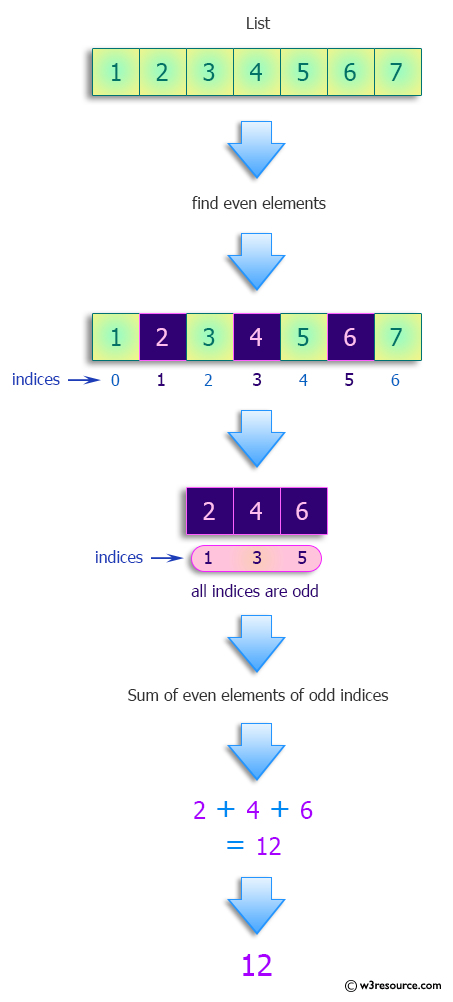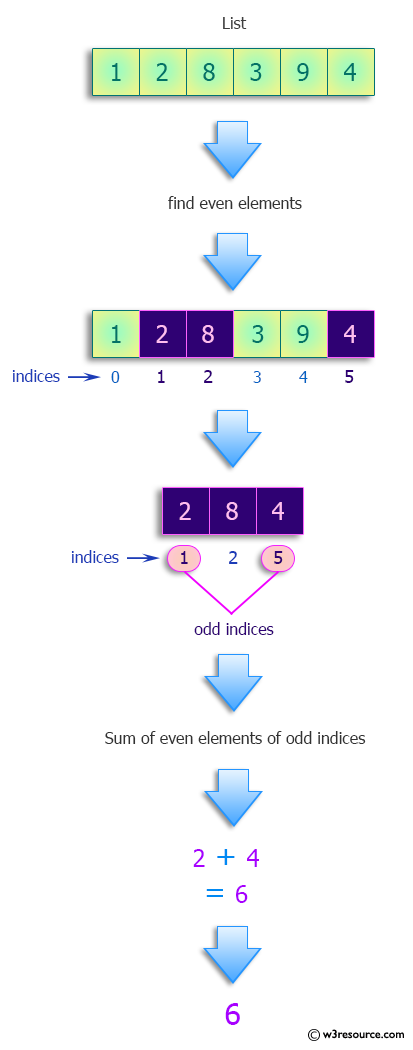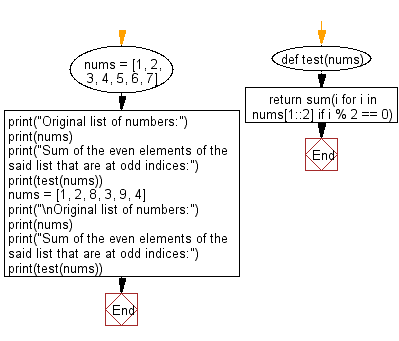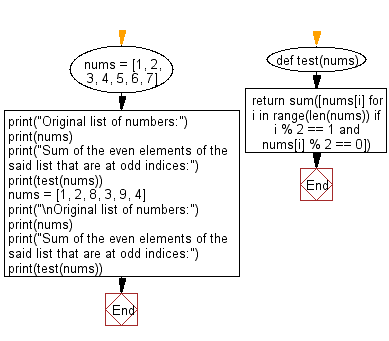﻿ Python: Find the sum of the even elements that are at odd indices in a given list - w3resource# Python: Find the sum of the even elements that are at odd indices

## Python Programming Puzzles: Exercise-63 with Solution

Write a Python program to find the sum of the even elements that are at odd indices in a given list.

```Input:
[1, 2, 3, 4, 5, 6, 7]
Output:
12

Input:
[1, 2, 8, 3, 9, 4]
Output:
6
```

Pictorial Presentation:Sample Solution-1:

Python Code:

``````#License: https://bit.ly/3oLErEI

def test(nums):
return sum(i for i in nums[1::2] if i % 2 == 0)
nums = [1, 2, 3, 4, 5, 6, 7]
print("Original list of numbers:")
print(nums)
print("Sum of the even elements of the said list that are at odd indices:")
print(test(nums))
nums = [1, 2, 8, 3, 9, 4]
print("\nOriginal list of numbers:")
print(nums)
print("Sum of the even elements of the said list that are at odd indices:")
print(test(nums))
``````

Sample Output:

```Original list of numbers:
[1, 2, 3, 4, 5, 6, 7]
Sum of the even elements of the said list that are at odd indices:
12

Original list of numbers:
[1, 2, 8, 3, 9, 4]
Sum of the even elements of the said list that are at odd indices:
6
```

Flowchart:## Visualize Python code execution:

The following tool visualize what the computer is doing step-by-step as it executes the said program:

Sample Solution-2:

Python Code:

``````def test(nums):
return sum([nums[i] for i in range(len(nums)) if i % 2 == 1 and nums[i] % 2 == 0])
nums = [1, 2, 3, 4, 5, 6, 7]
print("Original list of numbers:")
print(nums)
print("Sum of the even elements of the said list that are at odd indices:")
print(test(nums))
nums = [1, 2, 8, 3, 9, 4]
print("\nOriginal list of numbers:")
print(nums)
print("Sum of the even elements of the said list that are at odd indices:")
print(test(nums))
``````

Sample Output:

```Original list of numbers:
[1, 2, 3, 4, 5, 6, 7]
Sum of the even elements of the said list that are at odd indices:
12

Original list of numbers:
[1, 2, 8, 3, 9, 4]
Sum of the even elements of the said list that are at odd indices:
6
```

Flowchart:## Visualize Python code execution:

The following tool visualize what the computer is doing step-by-step as it executes the said program:

Python Code Editor :

Have another way to solve this solution? Contribute your code (and comments) through Disqus.

What is the difficulty level of this exercise?

Test your Programming skills with w3resource's quiz.

﻿

## Python: Tips of the Day

Clamps num within the inclusive range specified by the boundary values x and y:

Example:

```def tips_clamp_num(num,x,y):
return max(min(num, max(x, y)), min(x, y))
print(tips_clamp_num(2, 4, 6))
print(tips_clamp_num(1, -1, -6))
```

Output:

```4
-1
```